# Non-Terminating Combinator Expressions

S combinator expressions of leaf counts 1 through 10 that do not halt

## Details

The data is calculated by enumerating S combinator expressions of a certain leaf count using EnumerateCombinators and then applying SCombinatorHaltsQ to check if each expression's evolution will terminate.
The default content is a Dataset where the rows are labeled by the leaf count of the S combinator expression and contain the combinator expressions that do not terminate.

## Examples

### Basic Examples

Get the list of non-terminating S combinator expressions of leaf count 7:

 In:=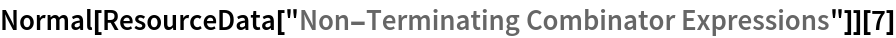Out=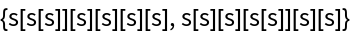Get the length of the list of non-terminating S combinator expressions of leaf count 10:

 In:=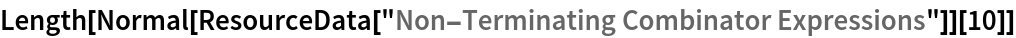Out=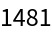The function SNT can be used to extend the dataset; however, note that as the leaf count for the combinator expressions increases, this computation will become increasingly more time-expensive:

 In:=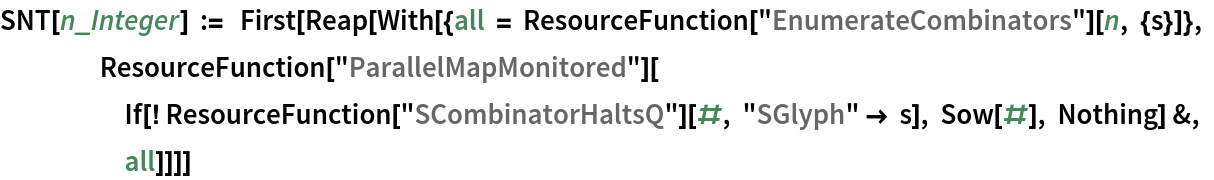Return the time required to calculate non-terminating S combinator expressions of leaf count 11:

 In:=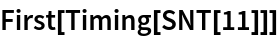Out=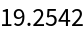Group terminating S combinator expressions of leaf count 7 in equivalence groups designated by their fixed point form:

 In:=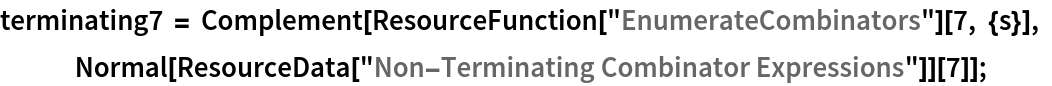In:=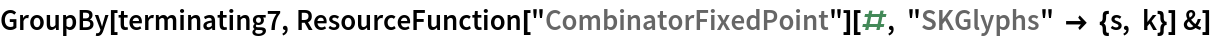Out=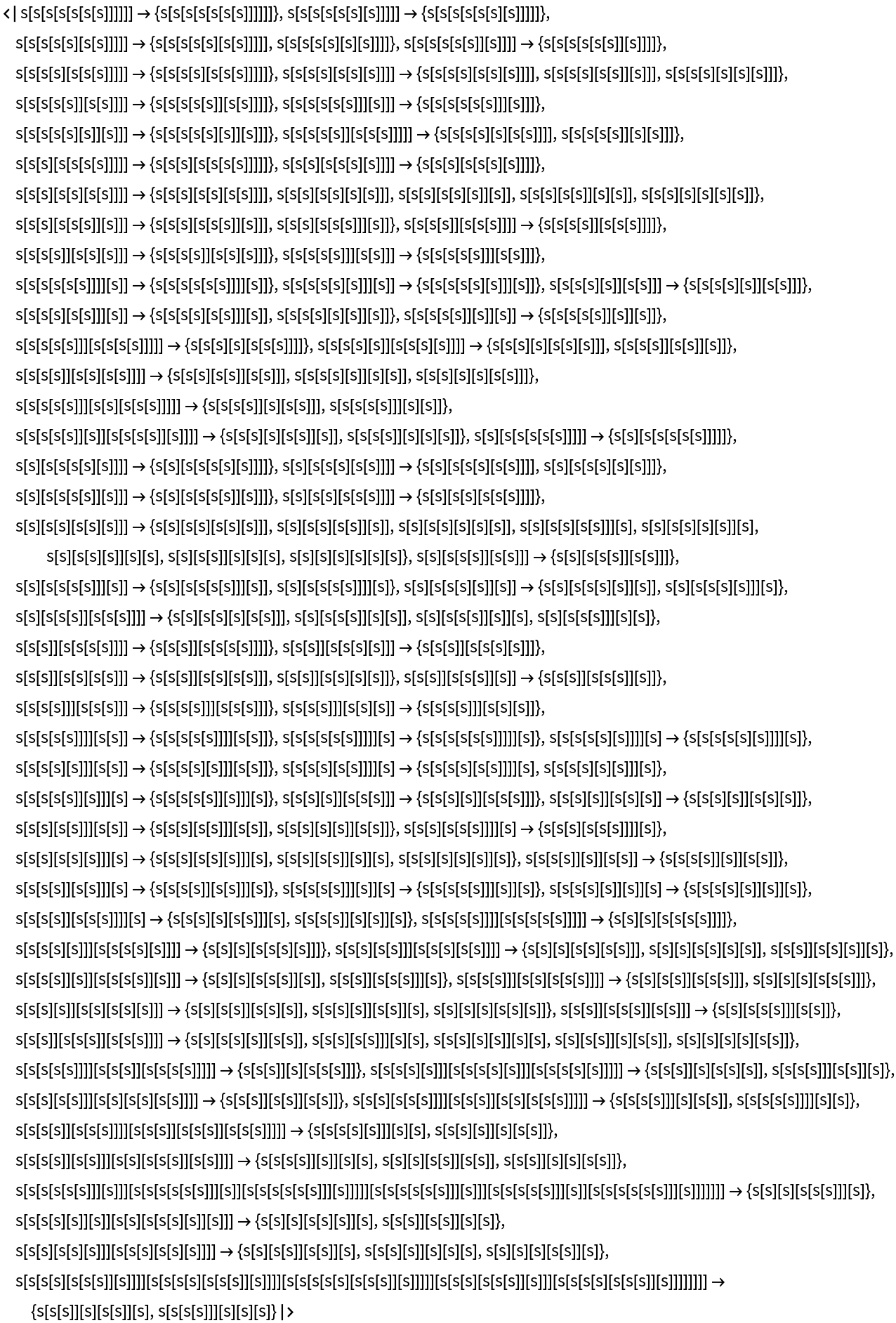Find the distribution of fixed point leaf counts for terminating S combinator expressions of leaf count 7:

 In:=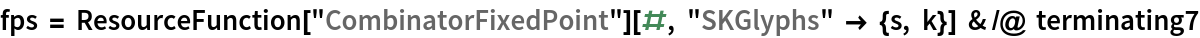Out=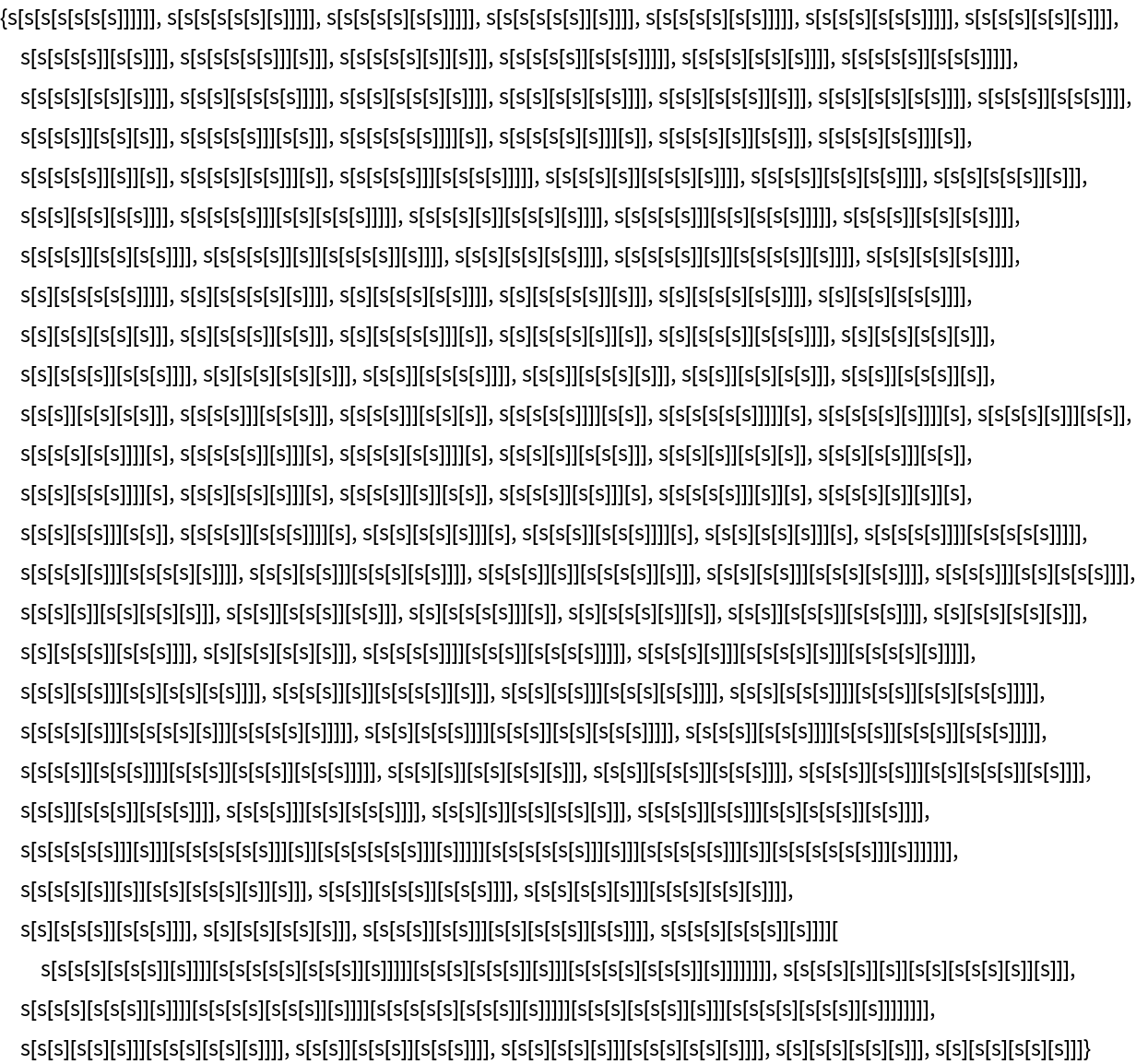In:=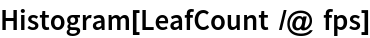Out=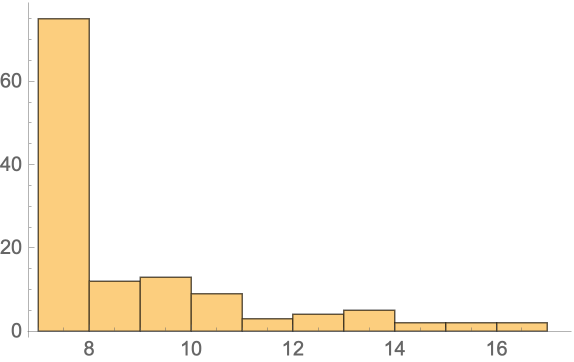Wolfram Research, "Non-Terminating Combinator Expressions" from the Wolfram Data Repository (2021)